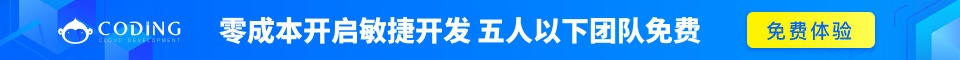# PHP array_uintersect_uassoc() 函数完整的 PHP Array 参考手册

### 实例

<?php
function myfunction_key(\$a,\$b)
{
if (\$a===\$b)
{
return 0;
}
return (\$a>\$b)?1:-1;
}

function myfunction_value(\$a,\$b)
{
if (\$a===\$b)
{
return 0;
}
return (\$a>\$b)?1:-1;
}

\$a1=array("a"=>"red","b"=>"green","c"=>"blue");
\$a2=array("a"=>"red","b"=>"green","c"=>"green");

\$result=array_uintersect_uassoc(\$a1,\$a2,"myfunction_key","myfunction_value");
print_r(\$result);
?>

## 定义和用法

array_uintersect_uassoc() 函数用于比较两个（或更多个）数组的键名和键值，并返回交集。

## 语法

array_uintersect_uassoc(array1,array2,array3...,myfunction_key,myfunction_value)

array1必需。与其他数组进行比较的第一个数组。
array2必需。与第一个数组进行比较的数组。
array3,...可选。与第一个数组进行比较的其他数组。
myfunction_key必需。用于比较数组键名的用户自定义函数的名称。

myfunction_value必需。用于比较数组键值的用户自定义函数的名称。

## 技术细节

返回值： 返回一个交集数组，该数组包括了所有在被比较的数组（array1）中，同时也在任何其他参数数组（array2 或 array3 等等）中的键名和键值。 5+完整的 PHP Array 参考手册